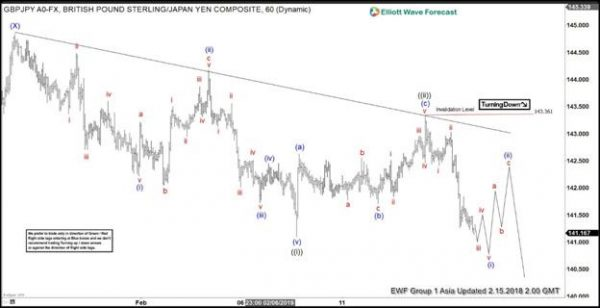Short term Elliott wave view in GBPJPY favors further downside due to the 5 swing sequence from Jan 26 high (144.84). We are calling the structure of the decline from 144.84 as an Impulse Elliott Wave. An impulse is a 5 waves move where subdivision of wave 1, 3, and 5 is also in 5 waves but in a lesser degree. Down from 144.84, wave ((i)) ended at 141.1 and wave ((ii)) ended at 143.35. Pair has broken below wave ((i)) at 141.1, signalling that the next leg lower has started.

We can see subdivision of wave ((i)) in impulsive 5 waves of a lesser degree. Wave (i) ended at 142.22, wave (ii) ended at 144.17, wave (iii) ended at 141.73, wave (iv) ended at 142.45, and wave (v) of ((i)) ended at 141.1. The internal of wave ((ii)) rally unfolded as a zigzag Elliott Wave structure. Wave (a) ended at 142.63, wave (b) ended at 141.7, and wave (c) of ((ii)) ended at 143.35. Subsequent decline in wave ((iii)) appears impulsive and we expect wave (i) of ((iii)) to end soon. Pair should then rally in wave (ii) to correct the decline from 143.35 high, but the rally should fail in 3, 7, or 11 swing below 143.35 for further downside. We don’t like buying the pair.

GBPJPY 1 Hour Elliott Wave Chart